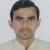true

Take Class 12 Tuition from the Best Tutors

•Affordable fees
•1-1 or Group class
•Flexible Timings
•Verified Tutors

Search in

# Law of conservation of angular momentum.Om Prakash Mishra
21/09/2018 00

It states that if net torque acting on an isolated system is zero, the angular momentum remains conserved.

Since Torque is equal to the rate of change of angular momentum so when torque is zero,

dL/dt=0

So, L= constant.

It is the very fundamental law of physics law of physics which helps to calculate angular momentum, torque, force, a moment of Inertia of a particle or rigid body and other variables related to it.

Sometimes no torque acts.

Sometimes anticlockwise torque= Clockwise torque, in both the cases law is applicable.

But the system should be decided with the utmost care.

0 Dislike
Follow 2## Other Lessons for You

Arithmetic Progression Example
Arithmetic Progression Example Q: The sums of n terms of two arithmetic progressions are in the ratio 5n+4:9n+6. Find the ratio of their 18th terms. ...Introduction to physics
Hello everyone, In these lesson we are learn about introduction of phyics. I give you first of all. a Example if we want to play any game we should know rules of that game that's like if...
S

### Looking for Class 12 Tuition ?

Learn from Best Tutors on UrbanPro.

Are you a Tutor or Training Institute?

Join UrbanPro Today to find students near you

X

### Looking for Class 12 Tuition Classes?

The best tutors for Class 12 Tuition Classes are on UrbanPro

• Select the best Tutor
• Book & Attend a Free Demo
• Pay and start Learning### Take Class 12 Tuition with the Best Tutors

The best Tutors for Class 12 Tuition Classes are on UrbanPro